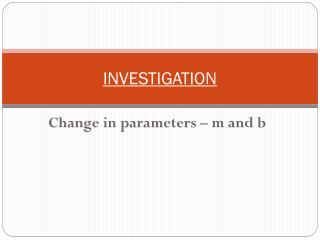DownloadDownload PresentationINVESTIGATION

# INVESTIGATION

Download Presentation## INVESTIGATION

- - - - - - - - - - - - - - - - - - - - - - - - - - - E N D - - - - - - - - - - - - - - - - - - - - - - - - - - -
##### Presentation Transcript

1. INVESTIGATION Change in parameters – m and b

2. INVESTIGATION • In this investigation, we will see how changes to the slope (m) and y-intercept (b) of a linear relation affects the graph of the line.

3. CHANGES TO THE SLOPE (m) OF A LINE 1. Graph the following lines on the TI-84 Plus graphing calculator. y = x y = 2x y = 5x Describe what happens to the steepness of the lines asthe value of the slope increases…

4. CHANGES TO THE SLOPE (m) OF A LINE 2. Graph the following lines on the TI-84 Plus graphing calculator. y = x y = ½x y = ¼x Describe what happens to the steepness of the lines as the value of the slope decreases…

5. In general: • As the value of the slope (m) increases, the line gets _________.  • As the value of the slope (m) decreases, the line gets ________________________. steeper flatter - closer to the x axis

6. CHANGES TO THE Y-INTERCEPT (b) OF A LINE 1. Graph the following lines on the TI-84 Plus graphing calculator. y = x y = x + 1 y = x + 4 Describe what happens to the graphs of the lines asthe value of the y-intercept increases.

7. CHANGES TO THE Y-INTERCEPT (b) OF A LINE 2. Graph the following lines on the TI-84 Plus graphing calculator. y = x y = x – 1 y = x – 3 Describe what happens to the graphs of the lines as the value of the y-intercept decreases.

8. In general: • As the value of the y-intercept (b) increases, the line moves _________. • As the value of the y-intercept (b) decreases, the line moves _________. up down